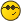# Tetration Forum

Full Version: Question about infinite tetrate
You're currently viewing a stripped down version of our content. View the full version with proper formatting.
probably a stupid question

the complex function h(z) = z^z^z^z^z^....

converges in a heart shaped region on the complex plane.

what is the area of that region ?

sorry
tommy1729 Wrote:what is the area of that region?

That's not a stupid question, its actually a good question, and I don't know the answer. But let's see if we can answer that using what is known about this region. Galidakis (1) and I (2) both call it the Shell-Thron region since these two authors have both investigated this region in great detail. Shell and Thron note that $${}^{\infty}b$$ converges where $$b=h^{1/h}$$ and $$|\ln(h)| \le 1$$ (a result which they both attribute to Barrow). So if we want the outer path, then we change the less-than sign to an equals sign, and this should give us the answer. If $$|\ln(h)| = 1$$, then we can parameterize this as $$\ln(h) = e^{it}$$ where $$i=\sqrt{-1}$$. This means that $$h = e^{e^{it}}$$, and putting this back in the relationship with b gives the parameterization
$$f(t) = {\left(e \uparrow e^{it}\right)} \uparrow {\left(e \uparrow {-e^{it}}\right)}$$
where $$a \uparrow b = a^b$$.

There are some interesting points that can be expressed with this function
$$\begin{tabular}{rrl} f(0) = & 1.4446678610097661337 & = e^{1/e} \\ f(1.4488307492834293737) = & 2.0477905274644031305 & + i\ 0.842045503530840715 \\ f(1.927907601568660839= & 0.8152027425068848021 & + i\ 2.0166402199122300356 \\ f(2.316910654383280043= & -0.380979728647791984 & + i\ 0.8997001955459000918 \\ f(\pi) = & 0.0659880358453125371 & = e^{-e} \end{tabular}$$

While we could use these points to integrate each section of the region, we could also use the parametric integration formula
$$A = \int_{x=\alpha}^{x=\beta} y dx = \int_{t=a}^{t=b} y(t) x'(t) dt$$
where $$x(t) = \text{Re}(f(t))$$ and $$y(t) = \text{Im}(f(t))$$. Using numerical integration we find that
$$\begin{tabular}{rl} A & = \int_{t=\pi}^{t=0} \text{Im}(f(t)) \left(\text{Re}(f(t))\right)^{'} dt \\ & = \frac{i}{2} \int_{h=1/e}^{h=e} h^{(1/h - 2)} \left(h^{1/h} - e^{1/(e^{1/\ln(h)} \ln(h))}\right) (\ln(h)-1) dh \\ & \approx 4.02546664 \end{tabular}$$
which is only the top half of the region, and since A = 4.02546664046975481171259768713, then
2A = 8.05093328093950962342519537425 should be the area of the whole region.

Andrew Robbins

(1) I.N.Galidakis The Birth of the Infinite Tetration Fractal.
(2) A.Robbins and H.Trappmann Tetration Reference, page 37.
I also made a pretty graph:
[attachment=469]
where the points are expressed with the previous parameterization:
f(2.8104771698906146985) = 0 + (...)i
f(2.316910654383280043-- minimum real part
f(2.2893404841466060077) = (...) +i
f(2.1025947819458660569) = 0 + (...)i
f(1.9681078867187501493) = (...) + 2i
f(1.927907601568660839-- maximum imaginary part
f(1.8909946364512036029) = 1 + (...)i
f(1.8869709745663253961) = (...) + 2i
f(1.5600433372731975189) = 2 + (...)i
f(1.5064318596452087082) = (...) + i
f(1.4488307492834293737) -- maximum real part
f(1.3099015915373765040) = 2 + (...)i

from this graph you can almost see how the area is almost 4, because it takes up almost 4 full squares, then it has some over-hand outside of these 4 squares, and the overhang looks like it could fill up the remaining area...

Andrew Robbins
andydude Wrote:
tommy1729 Wrote:what is the area of that region?

That's not a stupid question, its actually a good question, and I don't know the answer. But let's see if we can answer that using what is known about this region. Galidakis (1) and I (2) both call it the Shell-Thron region since these two authors have both investigated this region in great detail. Shell and Thron note that $${}^{\infty}b$$ converges where $$b=h^{1/h}$$ and $$|\ln(h)| \le 1$$ (a result which they both attribute to Barrow). So if we want the outer path, then we change the less-than sign to an equals sign, and this should give us the answer. If $$|\ln(h)| = 1$$, then we can parameterize this as $$\ln(h) = e^{it}$$ where $$i=\sqrt{-1}$$. This means that $$h = e^{e^{it}}$$, and putting this back in the relationship with b gives the parameterization
$$f(t) = {\left(e \uparrow e^{it}\right)} \uparrow {\left(e \uparrow {-e^{it}}\right)}$$
where $$a \uparrow b = a^b$$.

hmmm i was thinking about contour integration to arrive at a closed form ....
tommy1729 Wrote:hmmm i was thinking about contour integration to arrive at a closed form ....

Well if you can find a closed form, then more power to you! I give up.

Andrew Robbins